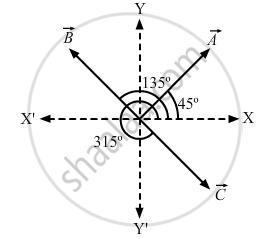Department of Pre-University Education, KarnatakaPUC Karnataka Science Class 11

# Add Vectors → a , → B and → C Each Having Magnitude of 100 Unit and Inclined to the X-axis at Angles 45°, 135° and 315° Respectively. - Physics

Add vectors $\vec{A} , \vec{B} \text { and } \vec{C}$  each having magnitude of 100 unit and inclined to the X-axis at angles 45°, 135° and 315° respectively.

#### Solution

First, we will find the components of the vector along the x-axis and y-axis. Then we will find the resultant x and y-components.
x-component of $\vec{A} = \ A\ cos \ 45^\circ =100 \cos 45^\circ = \frac{100}{\sqrt{2}} \text { unit }$

x-component of $\vec{B} = \vec{B} \cos 135^\circ = - \frac{100}{\sqrt{2}}$

x-component of $\vec{C}$ = $\vec{C}$ cos 315$^\circ$

= 100 cos 315°

$= 100 \cos 45^\circ = \frac{100}{\sqrt{2}}$
Resultant x-component $= \frac{100}{\sqrt{2}} - \frac{100}{\sqrt{2}} + \frac{100}{\sqrt{2}} = \frac{100}{\sqrt{2}}$Now, y-component of $\vec{A} = 100 \sin 45^\circ = \frac{100}{\sqrt{2}}$
y-component of $\vec{B} = 100 \sin 135^\circ = \frac{100}{\sqrt{2}}$
y-component of $\vec{C} = 100 \sin 315^\circ = - \frac{100}{\sqrt{2}}$
Resultant y-component
$= \frac{100}{\sqrt{2}} + \frac{100}{\sqrt{2}} - \frac{100}{\sqrt{2}} = \frac{100}{\sqrt{2}}$
Magnitude of the resultant $= \sqrt{\left( \frac{100}{\sqrt{2}} \right)^2 + \left( \frac{100}{\sqrt{2}} \right)^2}$

$= \sqrt{10000} = 100$
Angle made by the resultant vector with the x-axis is given by

$\tan \alpha = \frac{\text { y comp}}{\text { x comp }}$

$= \frac{100\sqrt{2}}{100\sqrt{2}} = 1$

⇒ α = tan−1 (1) = 45°

∴ The magnitude of the resultant vector is 100 units and it makes an angle of 45° with the x-axis.

Concept: What is Physics?
Is there an error in this question or solution?
Chapter 2: Physics and Mathematics - Exercise [Page 29]

#### APPEARS IN

HC Verma Class 11, Class 12 Concepts of Physics Vol. 1
Chapter 2 Physics and Mathematics
Exercise | Q 3 | Page 29
Share